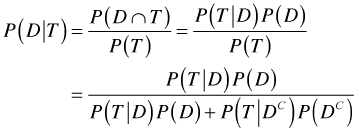# Question 10

• The type I error is the error of rejecting the null hypothesis when the null hypothesis is true.# Question 13• The right-tailed test is used. So there is a 5 percent area in the rejection region in the right tail of the sampling distribution. If we construct a 90 percent confidence interval, then the upper confidence limit will match the critical value. If the test of hypothesis is rejected at a 5 percent level of significance, then the test statistic fell in the rejection region. In other words, the hypothesized value of mean did not belong to the 90 percent confidence interval.

# Question 18

• A binomial model counts the number of successes out a fixed number of attempts at a task when each attempt has a constant probability of success# Question 20# Question 25

• The population of interest is the population you are trying to draw an inference about from the collected data sets.

# Question 31

• Response variable is about each sample, not the whole samples# Question 33

•• Notice the difference between sample mean and sample proportion# Question 37

• Equality of standard deviations is not necessary for a t-test to be valid. One of the conditions of a t-test is that the underlying populations must be normally distributed.